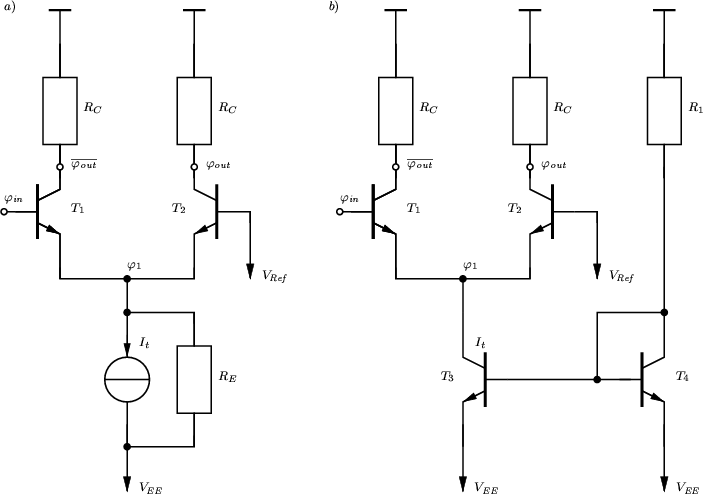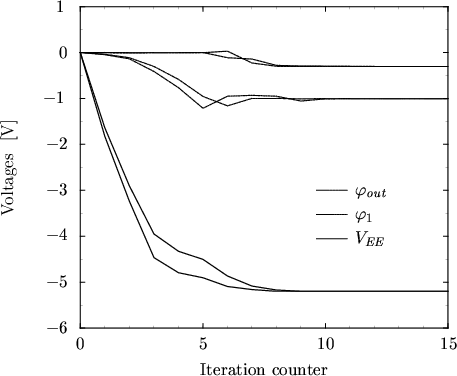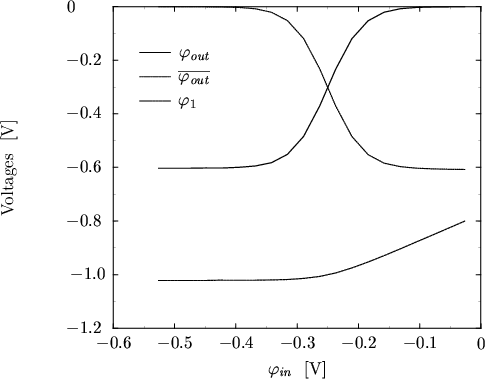Next: 6.3.3 CMOS Inverter Up: 6.3 Examples Previous: 6.3.1 Output Stage of

## 6.3.2 CML InverterThe schematic of a current mode logic (CML) inverter is shown in Fig. 6.12a. The evolution of the node voltages during DC operating point calculation is shown in Fig. 6.13. Best results were obtained with= 3. With ABC 20 iterations were needed whereas 24 with DBC.

For the other points following in the DC transfer characteristic the required number of iterations is exactly equal (12-18) and hence not shown. The simulated transfer characteristic is shown in Fig. 6.14.

For ABC one gets KA = 20/19 = 1.052 and for DBC KD = 24/21 = 1.1428 which both can be considered close to optimum.

In Fig. 6.12b the current source is realized as a current mirror. The number of unknowns increases to about 10000 and 48 iterations were needed using DBC and= 3. No convergence could be obtained for ABC.Next: 6.3.3 CMOS Inverter Up: 6.3 Examples Previous: 6.3.1 Output Stage of
Tibor Grasser
1999-05-31